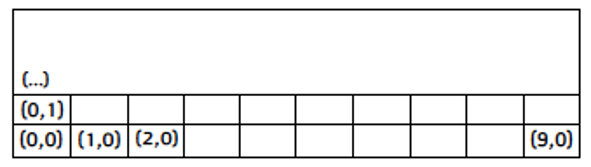# Using UDIM Values

Each patch represents one square unit in UV space; for instance, a square with dimensions 1x1. UDIM values are a way of representing the integer coordinates of that square, from the coordinates of its bottom-left corner in UV space.

Channels can contain up to ten patches across, and any number of patches upwards. This means that patches can have U indices between 0 and 9 inclusive, and V indices can be zero or any positive integer. With these values, the UDIM for a patch can be calculated using the formula:

udim = 1001 + u + (10 * v)

Note:  The U index limit of ten is currently hardwired.

The UDIM value of the bottom-left patch, which represents the UV space region (0,0) to (1,1), is 1001. The next patch to the right has a UDIM value of 1002, and the patch directly above the bottom-left is 1011. For example, the patch representing the UV space region (2,5) to (3,6) has a U index of 2 and a V of 5, so replacing the values in the formula, we get:

udim = 1001 + 2 + (10 * 5) = 1053